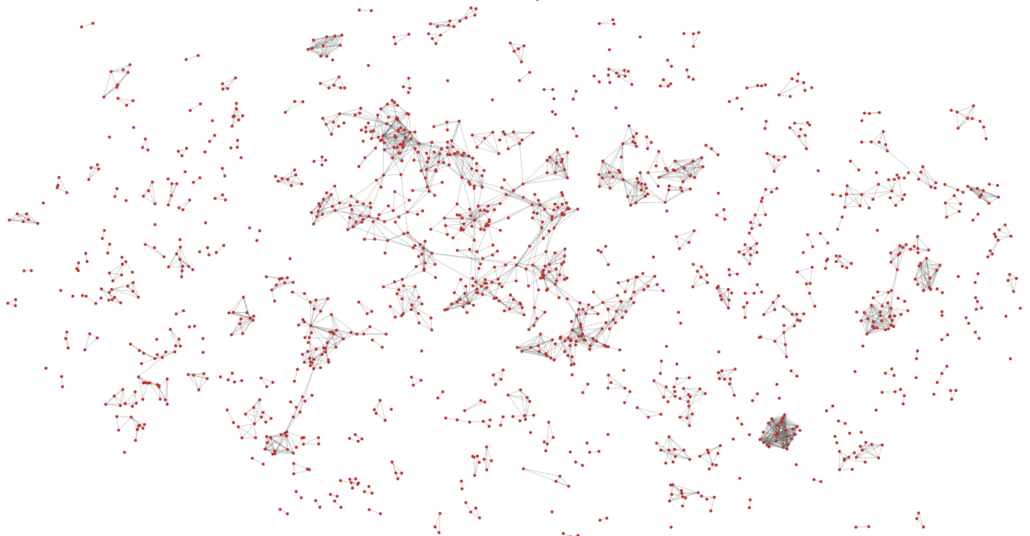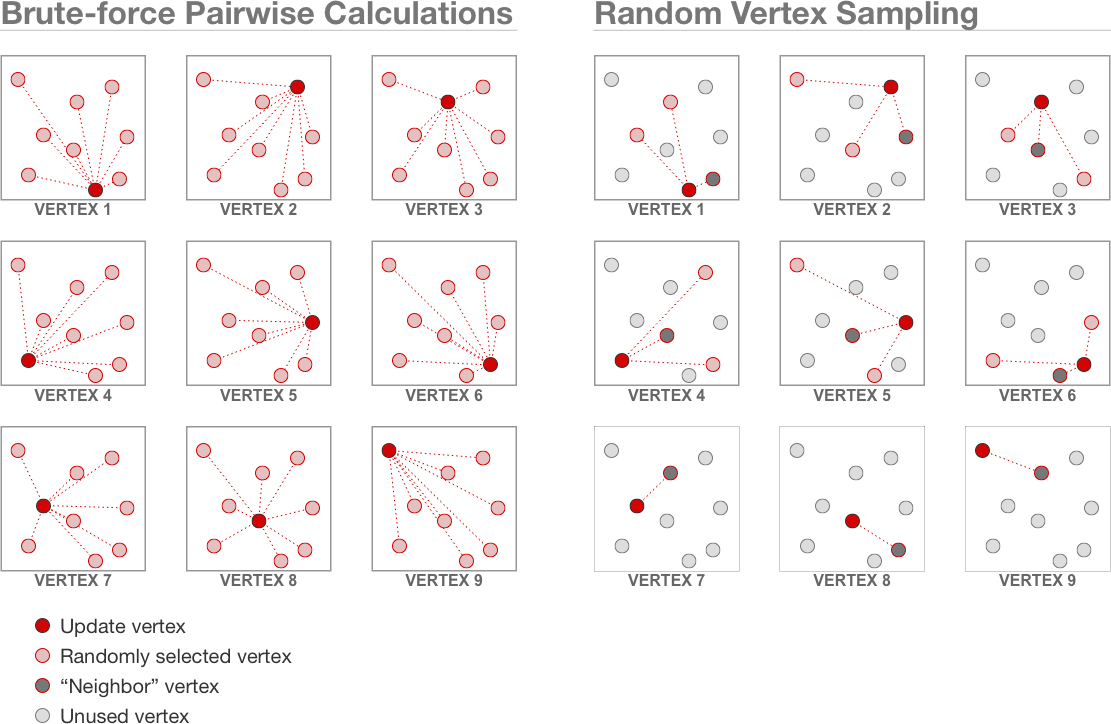What do you do if you need to visualize a large graph with hundreds of thousands or millions of vertices? Force-directed graph layouts are a good, general-purpose way to see the structure of a graph, but the algorithms can take several hours to run on such large graphs.

I propose a new way to speed up force-directed graph layout algorithms called Random Vertex Sampling. This is a simple approximation algorithm that runs in linear time while still producing good graph layouts! It also has lower memory requirements than other approximation algorithms like Barnes-Hut or Fast Multipole. I’m presenting a paper about Random Vertex Sampling this year at EuroVis, and I released an open source D3.js plugin.

## Random Vertex Sampling

Force-directed graph layout algorithms work by modeling the graph’s vertices as charged particles that repel each other and the graph’s edges as springs that try to maintain an ideal distance between connected vertices. The algorithms run an iterative physics simulation to find a good set of vertex positions that minimizes these forces.Comparison of the brute-force repulsive force algorithm and the Random Vertex Sampling algorithm. LEFT: The brute-force algorithm computes repulsive forces between each pair of vertices. RIGHT: Random Vertex Sampling uses a sliding window to select a subset of vertices to update. For each vertex in the update set, it randomly samples a smaller set of vertices to use for computing repulsive forces. Each vertex also has a small, constant-sized number of “neighbor” vertices that they use to compute repulsive forces. The algorithm is iterative, so after one iteration, the window slides forward and computes forces on a different subset of vertices.

In force-directed graph layouts, repulsive force calculations between the vertices are the main performance bottleneck. The brute-force algorithm computes repulsive forces between each pair of vertices, and therefore runs in O(n2) time at each iteration (n is the number of vertices in the graph).

Random Vertex Sampling reduces this runtime to O(n). It works by using a sliding window of length n¾ to select a subset of vertices to update their velocity. For each vertex in the update set, it randomly selects n¼ vertices to use for computing repulsive forces. Each vertex also has a constant-sized list of “neighbor” vertices used for computing repulsive forces on it. Because we choose the exponents carefully, and because the “neighbor” vertex lists are constant in size, the overall algorithm runs in linear time with O(n¾) auxiliary space requirements. Before the next iteration, the window slides forward n¾ positions in the vertex list to update a different set of vertices during the next iteration. Then the whole process repeats itself.

In contrast, consider tree-based approximation algorithms like Barnes-Hut or Fast Multipole. They build a spatial tree to approximate forces by aggregating distant vertices. This enables a O(n log n) runtime, which is pretty good, but still not as fast as Random Vertex Sampling. But, the spatial tree also comes with the expense of requiring O(n log n) auxiliary memory to store the tree, which is much more than Random Vertex Sampling.

## Faster and Just as Good!

In my experiments, I found that force-directed algorithms using Random Vertex Sampling run about 3 times faster on average than algorithms using Barnes-Hut. That’s a big improvement! Even better, it converges to a good layout faster, too.

I also found that Random Vertex Sampling produces layouts that are about the same quality as Barnes-Hut. Sometimes the layouts look a little more chaotic, though. We can improve this by first computing a layout using Random Vertex Sampling, and then running a few iterations of Barnes-Hut to smooth out the layout. That runs almost as fast as using Random Vertex Sampling by itself.

The detailed experiments use statistical analysis on five different graph layout quality metrics using a wide variety of graph types (social networks, transportation networks, geometric graphs, etc.). If you’re interested, see my research paper for all the details.